WEBINAR
Numerical Analysis of Magnetic Pulse Welding Process
Thursday, October 5, 2023
Time
SESSION 1
SESSION 2
CEST (GMT +2)
03:00 PM
08:00 PM
EDT (GMT -4)
09:00 AM
02:00 PM
HOME / Applications / Force on a Capacitor

# Force on a Capacitor

Used Tools:## Physics

Due to the charge they contain, capacitor plates (electrodes) are attracted to each other by an electric force. Magnitude of this force depends on the electric field inside the capacitor.

If the plate’s surface is much greater than the distance between them (), than the capacitor’s electric field can be regarded as homogenous and normal to the plates. In that case, field outside the capacitor is nearly zero, while the field intensity inside the capacitor receives equal contributions from both electrodes:(Figure 1). It is important to notice that the force acting on each electrode is proportional to the charge it stores and to the component of the electric field produced by the other electrode. For example, force on the positive plate is calculates as:(eq.1)
Potential difference between the electrodes (U) and the distance between the plates () define the field magnitude as. From equation 1 and the formula for capacitance of an air capacitor, the force acting on the capacitor plate can be expressed as:(eq.2)

whereis the permittivity of space. For plates with dimensions of 100 mm*100 mm at a distance d= 2 mm and a Voltage amplitude of 10 V    force magnitude is:.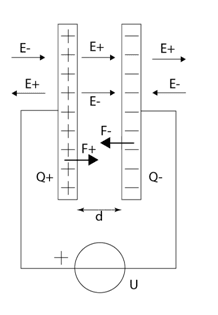Figure 1 - Parallel plate capacitor connected to a voltage

## Model

Model of capacitor with square shaped electrodes has been created in SOLIDWORKS. Surface of the plates is 100*100 mm2, while the distance between them is  2mm (Figure 2). Plates belong to the xy plane. The simulation is performed in the EMS Electrostatic study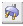. Copper is prescribed as a material to the electrodes, while air fills the space between and outside them.

To simulate potential difference prescribed to the capacitor by the voltage source, Fixed Voltage boundary condition should be prescribed to both electrodes.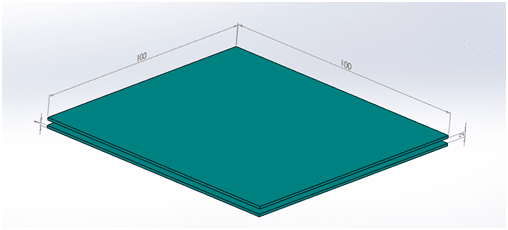Figure 2 - Solidworks model of capacitor

## Boundary Conditions

To account for the potential difference, a Fixed Voltage boundary condition is assigned to the both electrodes.
To do so,

1. In the EMS manger tree, Right-click on the Load/Restraint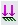folder.
2. Select Fixed Voltage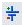.
3. Click inside the Bodies Selection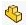box and then select the first  plate of the capacitor.
4. Type 0 in the Voltage box.
5. Click OK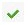.

For the second plate:

1. In the EMS manger tree, Right-click on the Load/Restraintfolder.
2. Select Fixed Voltage.
3. Click inside the Bodies Selectionbox and then select the second  plate of the capacitor.
4. Type 10 in the Voltage box.
5. Click OK.

## Results

1.     Under Results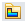, right click on the Electric Field folder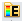and select 3D Fringe Plot.
The 3D Electric Field Property Manager Page appears.
2.     In the Section Clipping tab, select the plane for section clipping in which you want to inspect the field intensity.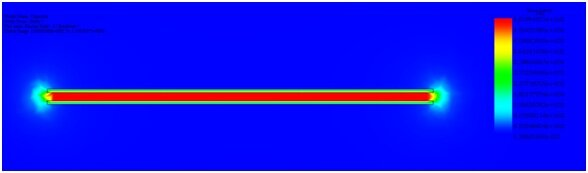Figure 3 - EMS results for electric field intensity

Electric field graph at Figure 3 shows that apart from field fringing at the boundaries of the plates, field is virtually non-existent outside the capacitor. Its intensity inside the capacitor is very uniform and it corresponds well to the predicated value of.
As expected, force practically exists only in the z axis, which is normal to the plates (Figure 4). Its intensity matches the analytical estimate within 3 %.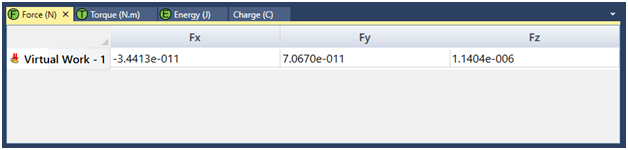Figure 4 - EMS results for force on the plate